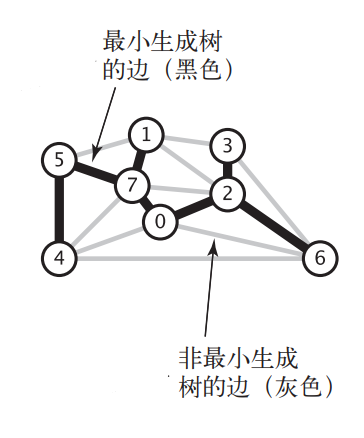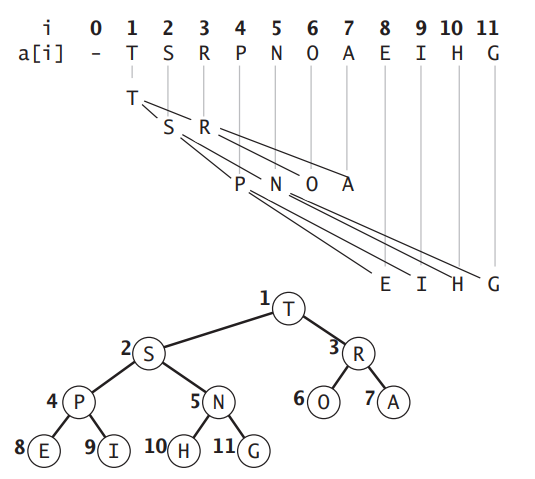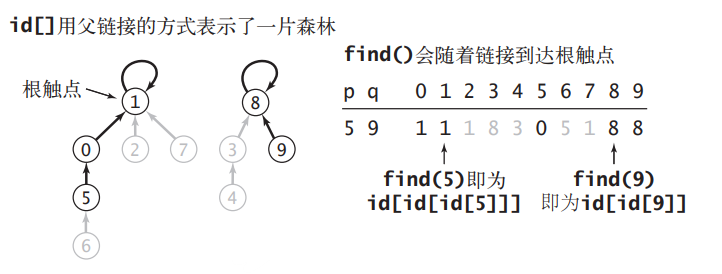# 如何在 Java 中实现最小生成树算法# 定义

$w(T)=\sum_{(u,v)\in T}w(u,v)$# 带权图的实现

《如何在 Java 中实现无向图》 中我们使用邻接表数组实现了无向图，其中邻接表上的每个节点的数据域只是一个整数，代表着一个顶点。为了方便最小生成树的迭代，我们将数据域换成 Edge 实例。Edge 有三个成员：顶点 v、顶点 w 和权重 weight，为了比较每一条边的权重，需要实现 Comparable 接口。代码如下所示：

package com.zhiyiyo.graph;

/**
* 图中的边
*/
public class Edge implements Comparable<Edge> {
private final int v, w;
private final double weight;

public Edge(int v, int w, double weight) {
this.v = v;
this.w = w;
this.weight = weight;
}

/**
* 返回边中的一个顶点
*/
int either() {
return v;
}

/**
* 返回边中的拎一个顶点
*
* @param v 顶点 v
* @return 另一个顶点
*/
int another(int v) {
if (this.v == v) {
return w;
} else if (w == v) {
return this.v;
} else {
throw new RuntimeException("边中不存在该顶点");
}
}

public double getWeight() {
return weight;
}

@Override
public String toString() {
return String.format("Edge{%d-%d %f}", v, w, weight);
}

@Override
public int compareTo(Edge edge) {
return Double.compare(weight, edge.weight);
}
}



package com.zhiyiyo.graph;

import com.zhiyiyo.collection.stack.Stack;

/**
* 带权无向图
*/
public class WeightedGraph {
private final int V;
protected int E;

public WeightedGraph(int V) {
this.V = V;
for (int i = 0; i < V; i++) {
}
}

public int V() {
return V;
}

public int E() {
return E;
}

int v = edge.either();
int w = edge.another(v);
E++;
}

}

/**
* 获取所有边
*/
public Iterable<Edge> edges() {
for (int v = 0; v < V; ++v) {
for (Edge edge : adj(v)) {
if (edge.another(v) > v) {
edges.push(edge);
}
}
}

return edges;
}
}



package com.zhiyiyo.graph;

/**
* 最小生成树
*/
public interface MST {
/**
* 获取最小生成树中的所有边
*/
Iterable<Edge> edges();

/**
* 获取最小生成树的权重
*/
double weight();
}


# Kruskal 算法## 二叉堆### API

package com.zhiyiyo.collection.queue;

public interface PriorQueue<T extends Comparable<T>> {
/**
* 向堆中插入一个元素
* @param item 插入的元素
*/
void insert(T item);

/**
* 弹出堆顶的元素
* @return 堆顶元素
*/
T pop();

/**
* 获取堆中的元素个数
*/
int size();

/**
* 堆是否为空
*/
boolean isEmpty();
}


### 插入package com.zhiyiyo.collection.queue;

import java.util.Arrays;

public class MinPriorQueue<T extends Comparable<T>> implements PriorQueue<T>{
private T[] array;
private int N;

public MinPriorQueue() {
this(3);
}

public MinPriorQueue(int maxSize) {
array = (T[]) new Comparable[maxSize + 1];
}

@Override
public boolean isEmpty() {
return N == 0;
}

@Override
public int size() {
return N;
}

@Override
public void insert(T item) {
array[++N] = item;
swim(N);
if (N == array.length - 1) resize(1 + 2 * N);
}

/**
* 元素上浮
*
* @param k 元素的索引
*/
private void swim(int k) {
while (k > 1 && less(k, k / 2)) {
swap(k, k / 2);
k /= 2;
}
}

private void swap(int a, int b) {
T tmp = array[a];
array[a] = array[b];
array[b] = tmp;
}

private boolean less(int a, int b) {
return array[a].compareTo(array[b]) < 0;
}

private void resize(int size) {
array = Arrays.copyOf(array, size);
}
}


### 删除最小元素@Override
public T pop() {
T item = array;
swap(1, N);
array[N--] = null;
sink(1);
if (N < (array.length - 1) / 4) resize((array.length - 1) / 2);
return item;
}

/**
* 元素下沉
*
* @param k 元素的索引
*/
private void sink(int k) {
while (2 * k <= N) {
int j = 2 * k;
// 检查是否有两个子节点
if (j < N && less(j + 1, j)) j++;
if (less(k, j)) break;
swap(k, j);
k = j;
}
}


## 并查集

### 判断是否连通package com.zhiyiyo.collection.tree;

public class UnionFind {
private int[] ids;
private int[] ranks;	// 每棵树的高度
private int N;			// 树的数量

public UnionFind(int N) {
this.N = N;
ids = new int[N];
ranks = new int[N];
for (int i = 0; i < N; i++) {
ids[i] = i;
ranks[i] = 1;
}
}

/**
* 获取连通分量个数
*
* @return 连通分量个数
*/
public int count() {
return N;
}

/**
* 获得连通分量的 id
*
* @param p 触点 id
* @return 连通分量 id
*/
public int find(int p) {
while (p != ids[p]) {
ids[p] = ids[ids[p]];   // 路径压缩
p = ids[p];
}
return p;
}

/**
* 判断两个触点是否连通
*
* @param p 触点 p 的 id
* @param q 触点 q 的 id
* @return 是否连通
*/
public boolean isConnected(int p, int q) {
return find(p) == find(q);
}
}


### 合并连通分量

• 如果 $$h_1 \neq h_2$$，新树高度会是 $$\max\{h_1, h_2\}$$
• 如果 $$h_1=h_2=c$$，新树高度会是 $$c+1$$

/**
* 如果两个触点不处于同一个连通分量中，则连接两个触点
*
* @param p 触点 p 的 id
* @param q 触点 q 的 id
*/
public void union(int p, int q) {
int pId = find(p);
int qId = find(q);
if (qId == pId) return;

// 将小树并到大树
if (ranks[qId] > ranks[pId]) {
ids[pId] = qId;
} else if (ranks[qId] < ranks[pId]) {
ids[qId] = pId;
} else {
ids[qId] = pId;
ranks[pId]++;
}

N--;
}


## 实现算法

package com.zhiyiyo.graph;

import com.zhiyiyo.collection.queue.MinPriorQueue;
import com.zhiyiyo.collection.queue.Queue;
import com.zhiyiyo.collection.tree.UnionFind;

import java.util.stream.Stream;
import java.util.stream.StreamSupport;

public class KruskalMST implements MST {
private Queue<Edge> mst;

public KruskalMST(WeightedGraph graph) {
UnionFind uf = new UnionFind(graph.V());

MinPriorQueue<Edge> pq = new MinPriorQueue<>();
for (Edge e : graph.edges()) {
pq.insert(e);
}

while (mst.size() < graph.V() - 1 && !pq.isEmpty()) {
Edge edge = pq.pop();
int v = edge.either();
int w = edge.another(v);
if (!uf.isConnected(v, w)) {
mst.enqueue(edge);
uf.union(v, w);
}
}
}

@Override
public Iterable<Edge> edges() {
return mst;
}

@Override
public double weight() {
Stream<Edge> stream = StreamSupport.stream(mst.spliterator(), false);
return stream.map(Edge::getWeight).reduce(0d, Double::sum);
}
}


# Prim 算法

Prim 算法的思想是初始化最小生成树为一个根节点 0，然后将根节点的所有邻边加入最小堆中，从最小堆中弹出最小的边 $$e_m$$，如果 $$e_m$$ 不会使得树中出现环，将将其并入树中。每当有新的节点 $$v$$ 被并入树中时，就得将 $$v$$ 的所有邻边加入最小堆中。重复上述过程直到 $$|T|=|V|-1$$，时间复杂度为 $$O(|E|\log|E|)$$。代码如下所示：

package com.zhiyiyo.graph;

import com.zhiyiyo.collection.queue.MinPriorQueue;
import com.zhiyiyo.collection.queue.Queue;

import java.util.stream.Stream;
import java.util.stream.StreamSupport;

/**
* 延时版本 Prim 算法
*/
public class PrimMST implements MST {
private boolean[] marked;
private MinPriorQueue<Edge> pq;
private Queue<Edge> mst;

public LazyPrimMST(WeightedGraph graph) {
marked = new boolean[graph.V()];
pq = new MinPriorQueue<>();

mark(graph, 0);
while (mst.size() < graph.V() - 1 && !pq.isEmpty()) {
Edge edge = pq.pop();
int v = edge.either();
int w = edge.another(v);

// 构成环则舍弃
if (marked[v] && marked[w]) continue;
mst.enqueue(edge);

if (!marked[v]) mark(graph, v);
else if (!marked[w]) mark(graph, w);
}
}

private void mark(WeightedGraph graph, int v) {
marked[v] = true;
for (Edge edge : graph.adj(v)) {
if (!marked[edge.another(v)]) {
pq.insert(edge);
}
}
}

@Override
public Iterable<Edge> edges() {
return mst;
}

@Override
public double weight() {
Stream<Edge> stream = StreamSupport.stream(mst.spliterator(), false);
return stream.map(Edge::getWeight).reduce(0d, Double::sum);
}
}